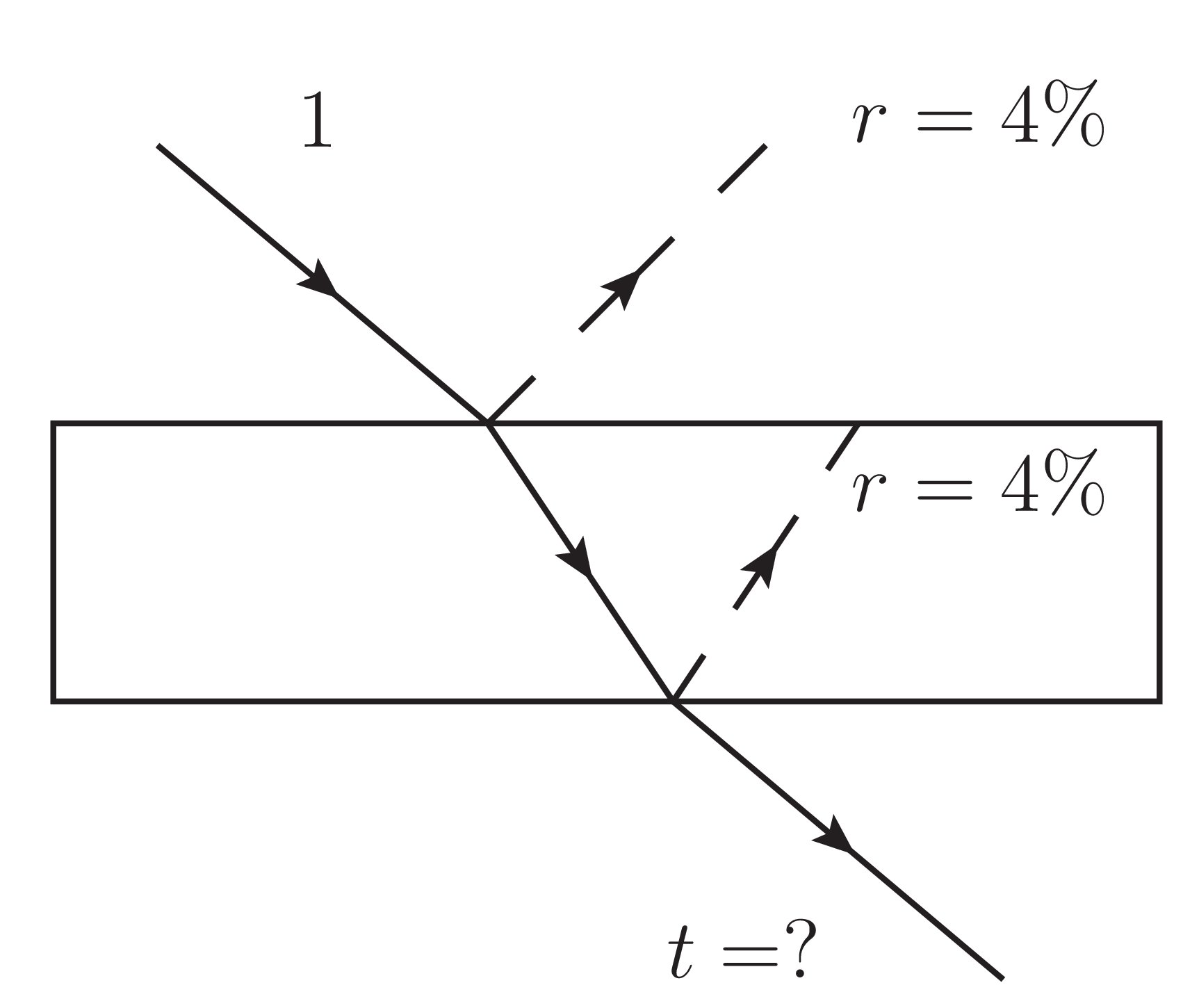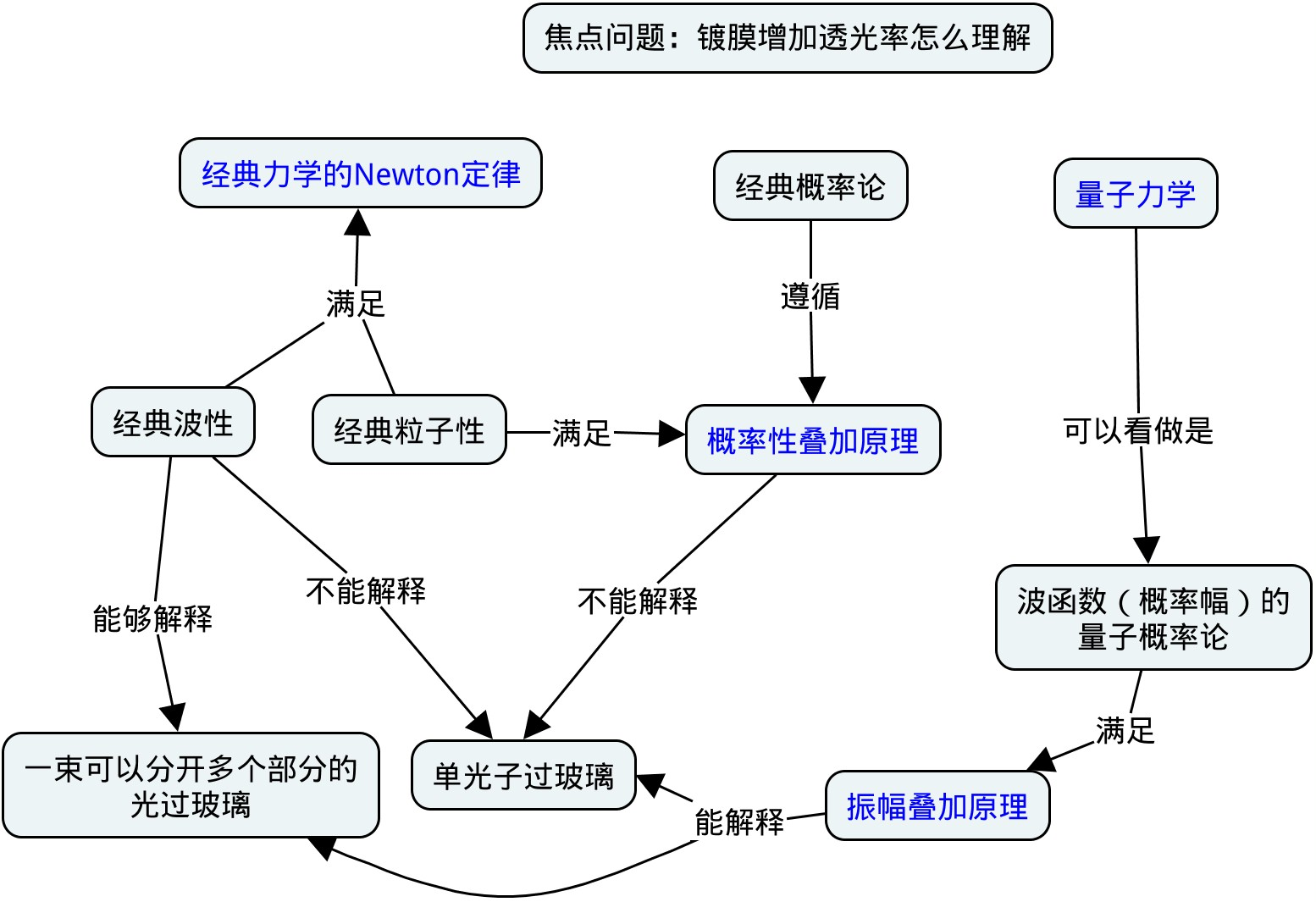# 光过玻璃是一件多么神奇的事情啊\begin{align}
\rho^{c} = p_{1} \left|1\right\rangle\left\langle 1\right| + p_{2} \left|2\right\rangle\left\langle 2\right| \\
\rho^{q} = \left(\sqrt{p_{1}} \left|1\right\rangle + \sqrt{p_{2}} \left|2\right\rangle\right)\left(\sqrt{p_{1}} \left\langle 1\right| + \sqrt{p_{2}} \left\langle 2\right|\right) \\
= p_{1} \left|1\right\rangle\left\langle 1\right| + p_{2} \left|2\right\rangle\left\langle 2\right| + \sqrt{p_{1}p_{2}}\left(\left|1\right\rangle\left\langle 2\right| + \left|2\right\rangle\left\langle 1\right|\right)
\end{align}